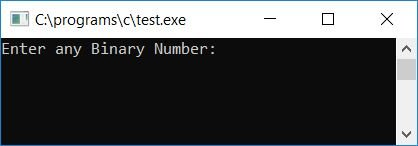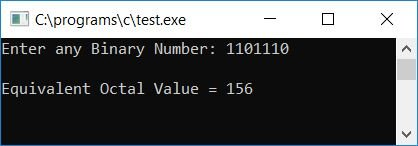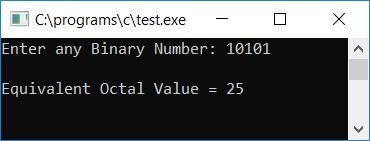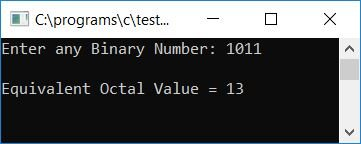# C Program to Convert Binary to Octal

In this article, we will learn how to create a program in C that can convert any given binary number (provided by the user at run-time) into its equivalent octal number. At last, we have also created a function named BinToOct() that does the same job.

But before going through the program, if you are not aware of

• Binary Number
• Octal Number
• Binary to Octal Conversion

then refer to the step-by-step process for binary to octal conversion. Now let's move on to the program.

## Binary to octal in C

The question is, "Write a program in C that converts any given binary number into its equivalent octal value." The answer to this question is:

```#include<stdio.h>
#include<conio.h>
int main()
{
int bin, oct=0, i=0, mul=1, count=1, rem, octnum;
printf("Enter any Binary Number: ");
scanf("%d", &bin);
while(bin!=0)
{
rem = bin%10;
oct = oct + (rem*mul);
if(count%3==0)
{
octnum[i] = oct;
mul = 1;
oct = 0;
count = 1;
i++;
}
else
{
mul = mul*2;
count++;
}
bin = bin/10;
}
if(count!=1)
octnum[i] = oct;
printf("\nEquivalent Octal Value = ");
for(i=i; i>=0; i--)
printf("%d", octnum[i]);
getch();
return 0;
}```

As the above program was written in the Code::Blocks IDE, here is the first snapshot of the sample run:Now supply any binary number, say 1101110, as input and press the ENTER key to see its equivalent octal value as shown in the second snapshot of the sample run given below:Here is the final snapshot of another sample run:#### Program Explained

• Receive any binary number from the user, say 1101110, at program runtime.
• Create a while loop that runs until the value of the binary number (bin) becomes 0.
• bin (1101110) is not equal 0 on the first run, so program flow continues inside the loop.
• And bin%10 or 1101110%10 or 0 gets initialized to rem, oct + (rem*mul) (we have initialized 0 to oct and 1 to mul at the start of the program) or 0 + (0*1) or 0 gets initialized to oct.
• We have initialized 1 to count at the start of the program, therefore at first run, the value of count is not equal to 3, therefore while dividing the value of count or 1 by 3, we will not get a remainder of 0, therefore program flow goes inside the else block (as the condition of the if statement evaluates to false at first run of the while loop), and inside the else block, mul*2 or 1*2 or 2 gets initialized to mul, and the value of count gets incremented and becomes 2.
• At last, bin/10, 1101110/10, or 110111 gets initialized to bin, and again the program flow goes back to the while loop's condition.
• Therefore, bin!=0 or 110111!=0 evaluates to true, so the program flow again goes inside the loop.
• And bin%10 or 110111%10 or 1 gets initialized to rem, and oct + (rem*mul) or 0 + (1*2) or 2 gets initialized to oct.
• Again, the condition of the if statement, that is, count%3==0 or 2%3==0, evaluates to false, therefore program flow goes inside the else block, and mul*2 or 2*2 or 4 gets initialized to mul, and the value of count gets incremented and becomes 3.
• At last, bin/10, 110111/10, or 11011 gets initialized to bin, and again program flow goes back to the loop's condition.
• Therefore, bin!=0 or 11011!=0 evaluates to true, so the program flow again goes inside the loop.
• And bin%10 or 11011%10 or 1 gets initialized to rem, and oct + (rem*mul) or 2 + (1*4) or 6 gets initialized to oct.
• Now the condition of the if statement, that is count%3==0 or 3%3==0, evaluates to true, therefore program flow goes inside the if block, and oct or 6 gets initialized to octnum[i] (we have initialized 0 to i at the start of the program) or octnum, and 1, 0, and 1 get initialized to mul, oct, and count, respectively. And the value of i gets incremented and becomes 1.
• At last, bin/10 or 11011/10 or 1101 gets initialized to bin, and again the program flow goes back to the loop's condition.
• Therefore, bin!=0 or 1101!=0 evaluates to true, and the program flow again goes inside the loop and follows the same procedure as described above.
• After exiting the while loop, check whether the value of count is not equal to 1 or not; if it is, then the value of oct gets initialized to the last index of the octnum[] array.
• Finally, print the value of octal digit one by one from the last index to the first index.

### Binary to Octal in C using a User-Defined Function

The question is, "Write a program in C that converts binary to octal using a user-defined function named BinToOct()." Here we have declared the variable i and the array octnum as global variables (out of both the functions) to make them known inside both the main() and BinToOct() functions.

```#include<stdio.h>
#include<conio.h>
void BinToOct(int bin);
int i=0;
int octnum;
int main()
{
int binnum;
printf("Enter any Binary Number: ");
scanf("%d", &binnum);
BinToOct(binnum);
printf("\nEquivalent Octal Value = ");
for(i=i; i>=0; i--)
printf("%d", octnum[i]);
getch();
return 0;
}
void BinToOct(int bin)
{
int oct=0, mul=1, count=1, rem;
while(bin!=0)
{
rem = bin%10;
oct = oct + (rem*mul);
if(count%3==0)
{
octnum[i] = oct;
mul = 1;
oct = 0;
count = 1;
i++;
}
else
{
mul = mul*2;
count++;
}
bin = bin/10;
}
if(count!=1)
octnum[i] = oct;
}```

Here is the final snapshot of the above program's sample run:#### The same program in different languages

C Quiz

« Previous Program Next Program »